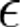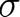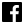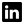# What bias and variance really are

16 Oct 2020

### In this article, I want to present intuition that stands behind bias-variance decomposition. We can see the process of learning from different perspectives. In machine learning, in general, we can see learning as a process leading us to find the best hyperplane that allows us to explain our problem. In this heuristic definition there are two aspects that are key to understanding the process: “the best” and “explain our problem”.

In any kind of learning, we have access only to some part of the information, so we can assume that the data we have can represent only some aspects of our problem. All this data always will be only a representation of some phenomenon, so we can intuitively feel that it will be somehow misled by different kinds of mistakes. Not only errors are limiting our information, but also the fact that you can rarely measure all ranges of values, especially in multidimensional problems.

Let’s notice that humans are perfect with learning from incomplete data. Imagine someone presenting to you a set of gate screws and ice screws, which you probably have never seen in your life but your capability of distinction between these two kinds of climbing gear grows from 0 accuracies to almost 1 in seconds. We must stress here that human training for this kind of classification is different from the training of artificial classifiers. People learn all the time so we have pre-trained classifiers and we use some kind of inference. We also master the process of quick retraining our classifiers.

Now, let’s leave these sophisticated neuro-tricks that our brain is using and focus on the problem of learning from real data in the machine learning framework. We have a few kinds of problems with real-world data. Size of data set, quality of data, and complexity of the phenomenon that we want to explain. It’s obvious that in the limit of an infinite data set some of these obstacles are no longer a problem but we are living in the real world so we must challenge real data sets. Let’s start our solution by suggesting some models and finding the best fit for them. Let’s use the following notation:

•– a set of observed outputs of the phenomenon that we want to model.
Example: male/female for gender classification. We can use some indexing here sowill be the value of the first object in the probe.
We assume that this observation depends onso we can write down:•– a set of variables that explain our phenomenon. In our example of gender classification, it can be height, weight, etc.
•– the model that we are trying to fit. All the knowledge that we want our model to gain is hidden in this part. So, using our gender example again here is an expertise that a person of 1.5 m height and 50 kg weight is probably a woman. Of course, generalization in the other cases happens also at this point. Generally, machine learning models are not memorizing examples, they are learning patterns from the data.
•– the difference between the model and observed representation.
This is an error that is connected to every measurement.
•– fitted estimator – this is all the knowledge that we were able to gain from the data set at a fixed step of learning.

•– the error function (tells how wrong we were), that is, it measures how good our predictive ability is. We should notice here that this is a relative measure, it depends on our data set.

### Rule standing behind ML

We can’t simply fit our hyperplane to this representation because every point has some error. Assuming that the exact coordinate of the point is the same as the coordinates of a real point in some problem space is not correct. Let’s use the following notation:

So, our goal is to find the function:

in this way that:

will have a minimum value for this specific choice.
Yup, this is machine learning explained in one sentence.

### Why is it worth to make friends with bias and variance

Let’s introduce one more idea (the reader must be familiar with expected value to fully understand this paragraph, read more: Expected_value).

Let’s say that we want somehow to check how far our estimationis from real values.
So let’s calculate the distance:But we are interested in average error so the proper measure will be an expectation of that expression:Let’s look a little closer on that guy:

Where:

And:is an irreducible error connected with the variance of noise in measure.But, the other two guys are more interesting. An estimator is a random variable so it has some probability distribution if a mass of this probability is close to the center we are unlikely to be wrong or if we are wrong we are only slightly incorrect. In analogy, let’s imagine that we are trying to learn to distinguish between circles and squares. We have some set of labeled examples and we are trying again and again after one epoch of training we will be very likely to be wrong on a new example. After a few epochs of training, we are better and better. In the end, we are the master of the circle/square classification. This process is a perfect representation of how our distribution was sharpening around the correct answer. In analogy when I’m learning something advanced like math or programming I have the feeling of uncertainty about new information. So variance has a perfect corresponding with the human process of learning., on the other hand, is the distance of real undisturbed function representing the problem and our estimation. So let’s assume that we want to estimate the weather on some fixed hour based on a few parameters perfectly measured in the previous hour e.g.: pressure, temperature, humidity, irradiation, etc.

We have some model:, we made a lot of measurements and we found the model parameters with the recipe above so the function:is minimal for these parameters. But let’s assume for clarity that the function that I take for my estimation is linear, so probably my Bias will be high, because it can’t capture the complexity of the weather forecast.

So, when we calculate Bias, we know how our model complexity corresponds to the phenomenon complexity. Of course, we want good correspondence, because a simple model can’t be a good estimation and too complex one will force more computational resources for training and will be likely to overfit.
Now, we can focus on using this intuition in real machine learning projects. This leads us to the method of evaluating models without statistical stuff like AIC, BIC, etc.

### How to use bias and variance in practice

In standard machine learning, we divide our data into two sets: training and test. Training is some kind of playground for the algorithm to learn and the test set is a real word. The human education system is also planned this way, you are learning by an academic example for further usage at work. The difference is in the assumption that the ML training set should be the best representation of the real world possible. We split our data into two sets and we start training and we have the following scenario:  86% accuracy on the test set and 99% accuracy on the training set. We can say that the model is advanced enough to capture complexity but the generalization is poor – this is called overfitting. We can connect bias with error on the training set and variance with error on the test set. In this example bias is low and variance is high, what we can do is add more data for lowering variance. We need to see more examples for better generalization.

Other ways for addressing variance can be adding regularization or early stopping, this way we will make our algorithm more noise-robust, but this will increase our bias. We can also reconsider our model if we can’t capture the real trend of the phenomenon; think if you can transform features some way. For example, if we know that our model has a logarithmic or exponential dependency on some features, we should do this transformation. That will lead us to a simple dynamic to capture by model.

Now let’s analyze the opposite example: 87% accuracy on the training set and 88% accuracy on the test set. In this case, the model is under fitted and it can’t capture the complexity of data. In this example bias is high and we need to use some more sophisticated model. So what we can do is to add some complexity to the model (layers, neurons, parameters, etc.). Note that adding more data will not help. You can also try to transform features or reduce regularization, but be careful with variance. As you can see this is a heuristic but a very useful one.

### How to make better decisions in the machine learning project?

In this article, we briefly introduce rules of thumb about an easy way of evaluating what we should do to achieve a correctly trained model without any advanced math methods. For huge projects, it’s crucial to decide what we are going to do with our model to improve its performance.
The best thing about it? This method can let you make better decisions by managing your machine learning projects without hardly any calculations.

Read more about Artificial Intelligence and Principles of machine learning in our previous blog post:

Artificial Intelligence – what is it and how to get to know it better?

For further reading, you can look here Pattern Recognition and Machine Learning and here Machine Learning Yearning.

Share on: